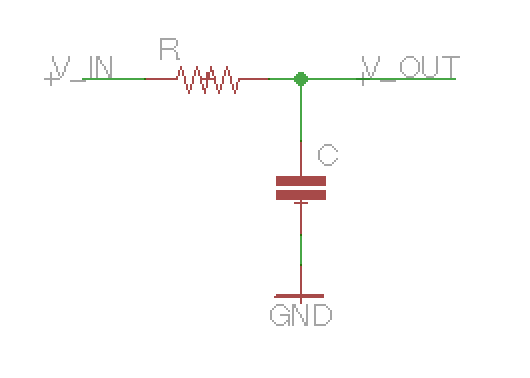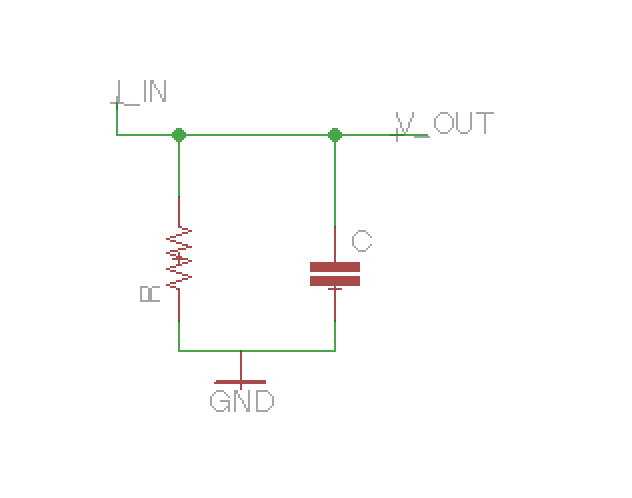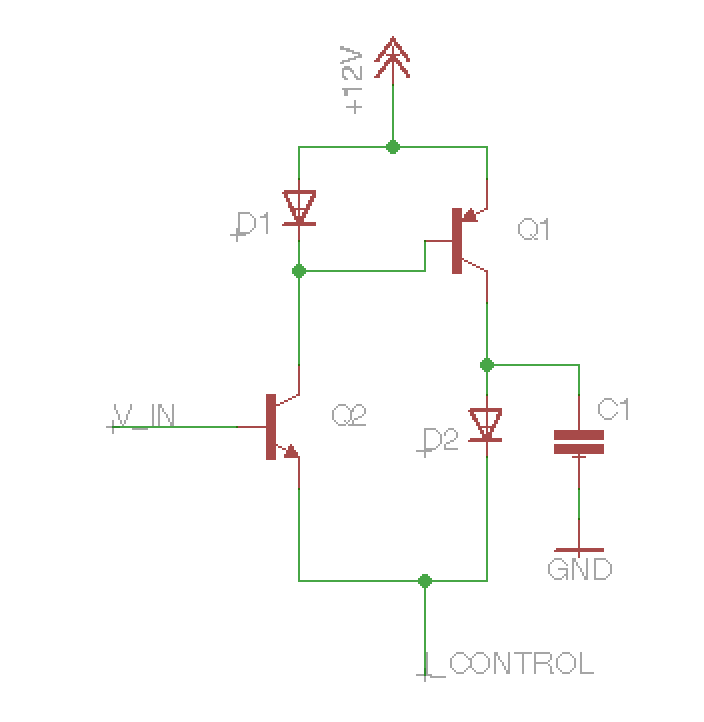# russell mcclellan

## musical hardware and software

russell.mcclellan@gmail.com

view as pdf

# Background

Back in the heyday of the giant analog polysynths (i.e. the early to mid 80s), most synthesizers were powered by analog integrated circuits. The small size of these chips is what enabled synths to have so many voices in such a relatively small amount of space. Generally, each standard synth function (VCO, VCF, VCA) was implemented by a dedicated chip.

At first glance, there's nothing incredibly extraordinary about the CEM3372 VCF chip. It is famous for its use in the wildly flexible Oberheim Xpander filter circuit, but the flexibility of that filter comes from a clever circuit trick, not from anything specific to a single chip . The CEM3372 also has an unusual number of "convenience features" - an integrated VCA for the output and two VCAs for voltage-controlled mixing on the input. Perhaps because of these extra features it was marketed as a "signal processor" chip instead of just a "filter".

However, a deeper look reveals that the filtering works through a slightly different technology than the previous generation of VCF chips (e.g. CEM3320, SSM2040, IR3109), and I thought it might be worth writing up how it works as the technology has applications for discrete circuits as well.

# Low Pass Filters

Low pass filters are designed to remove high frequency components from a signal. Any components higher in frequency than a certain "cut-off" frequency will be removed. Musically, filters are one of the most basic electronic "timbre modifiers" and are included in almost all synthesizers. In fact, the "sound" of the synthesizer is often defined by the character of its filter. Whole genres have sprung up exploiting the effect created from changing the cut-off frequency over time.

WARNING! The rest of this post will probably require some (hopefully basic) familiarity with circuits.

Perhaps the most basic lowpass filter consists of the following:This is a classic voltage divider, with one of the resistors replaced with a capacitor. In fact, we can use the concept of impedence to treat the capacitor as a sort of frequency-dependent resistor. For voltages that don't change, the capacitor acts as an open circuit; for high frequencies, it acts as a short; and for medium frequencies, somewhere in between. Just like a classic resistive voltage divider, when we put the capacitor in a divider the output voltage is:

$$V_{out} = V_{in}\frac{Z_{C}}{{Z_{C}}+{Z_{R}}}$$

which reduces to

$$V_{out} = V_{in}\frac{1}{1 + \frac{Z_R}{Z_C}}$$

If the voltage is constant, $$Z_C$$ will become infinite so $$V_{out} = V_{in}$$. If the voltage is high frequency, $$Z_C$$ will be zero so $$V_{out} = 0$$. The exact "cut-off" point turns out to be $$\frac{1}{2\pi RC}$$ - but to understand why you'll need some math outside the scope of this blog post.

While this voltage-in, voltage-out divider is the most common scheme for low pass filtering, there are of course many others. The way the CEM3372 works is by sending the signal through an input current rather than voltage, and then putting the resistor and capacitor in parallel:To analyze this arrangement, we use the familiar formula for parallel impedence:

$$V_{out}= I_{in}\frac{Z_RZ_C}{Z_C + Z_R}$$

or, after some alegbra

$$V_{out}= I_{in}Z_R\frac{1}{1 + \frac{Z_R}{Z_C}}$$

So, since $$Z_R$$ does not vary with frequency, this is the exact same sort of lowpass filter as the first one we considered. In synthesizer filters, unlike most analog circuits, you need to be able to change the cut-off point of the filter based on electrical signals. To do this, we need some way to electronically control either the resistance or the capacitance.

# The CEM3372 Circuit

As described in this patent, in the CEM3372, we simulate a current-controlled resistor by sending a large, steady current through a diode, and then sending a very small current as the signal. At a small enough signal current, the diode behaves as a voltage source followed by a resistor whose value is set by the large, steady current going through it. This is often called the diode's dynamic resistance.. This is approximately $$\frac{26mV}{I_{steady}}$$.

Since synthesizers generally send audio signals as voltages, the CEM3372 circuit also includes voltage-to-current converters for both the audio and control input. Here's the circuit with control signal's voltage-to-current converter not shown.The control signal is sent as a current $$I_{control}$$, and the audio input is $$V_{in}$$. D1 and Q1 comprise a common circuit idiom called a current mirror, which mirrors the current through D1 out the collector of Q1. In this case, with $$V_{in}$$ steady, both D1 and Q1 will have $$\frac{I_{control}}{2}$$ going through them.

Now, let's assume $$V_{in}$$ is quite small. Then increasing $$V_{in}$$ will create a small increase of the current through Q1's collector. Let's call this small-scale current $$i_{in}$$. Then this is mirrored by D1 and Q1, such that the same current as Q2's collector, i.e. $$\frac{I_{control}}{2}+i_{in}$$ appears at Q1's collector. Since $$\frac{I_{control}}{2}$$ is steady, none of it flows through C1, and it all goes through D2. Since the steady current is so much larger than $$i_{in}$$, we can treat D2 as a dynamic resistor with resistance set by the steady current. So, in practice, we now have a very similar situation to the parallel low pass filter considered above, with the input current being the small-scale $$i_{in}$$.

The rest of the post will be a slightly more detailed analysis of this situation. The main result here is the transfer function is more-or-less the same as the RC filter case, except the amplitude of the output does not depend on the resistance.

The main difference in the CEM3372 situation from the currentbased- RC filter above is that in the RC filter, both the resistance and the capacitor are tied to ground. That's not true here, as D2 goes accross $$V_{out}$$ to $$V_{in} - V_{Q2be}$$, where $$V_{Q2be}$$ is the base-emitter voltage of Q1. This acts as afeedback loop and eliminates the awkward effect of increasing the output amplitude when the resistance increases.

Let's recall first that for small signals, the diode acts as a resistor in series with a voltage source - call the voltage $$V_{D}$$, and the resistance $$Z_{D}$$. Since Q2 and D2 are matched, and the large-scale currents are the same, they have the same resistance  and voltage. So, the voltage accross D2 is given by $$V_{D} + i_{D2} Z_{D}$$, and $$V_{Q2be} = V_{D} + i_{in} Z_{D}$$

So, to sum up, we have a system of equations:

$$i_{D2} + i_{C1} = i_{in}$$ $$V_{D2} = V_{D} + i_{D2}Z_{D}$$ $$V_{Q2be} = V_{D} + i_{in}Z_{D}$$ $$V_{out} = V_{D2} + V_{in} - V_{Q2be} = i_{C1} Z_{C1}$$

which can be solved with basic algebra to

$$V_{out} = V_{in}\frac{1}{1 + \frac{Z_{D}}{Z_{C1}}}$$

as we were trying to show. Using the formula for an RC filter's frequency cut-off from above, we have

$$f_{c} = \frac{I_{control}}{4 C \pi \cdot 26mV}$$

which, as we hoped, is directly controllable from the control current.

Using a diode's dynamic resistance inside the differential pair arrangement provides many advantages over the standard OTA based low-pass filter. The main one is that in the CEM3372, unlike competitor chips, the stages themselves don't need to have feedback. This means far fewer external components and less trimming required. The signal is passed in as a voltage and comes out as a voltage, so the stages can be cascaded without ever converting from current to voltage .

The downside to all this is that you lose the ability to make a voltage-controlled integrator, which is a necessary part of the popular state variable filter used in the Oberheim SEM, Jupiter 6, and many other synthesizers.

# footnotes

1
Like many synthesizer-related tricks, its origin can be traced back to Bernie Hutchin's ElectroNotes newsletter.

2
Of course, experienced circuiteers will recognize that in addition to having an effect on the amplitude, capacitors also have an effect on the phase.s

3
Of course, for Q2, this is actually the so-called transimpedence, the base-emitter voltage change per amp of collector-emitter current. Base currents aren't considered in this quick-and-dirty analysis.

4
As mentioned in the patent, base currents will create errors that will have to be compensated when the stages are connected directly.